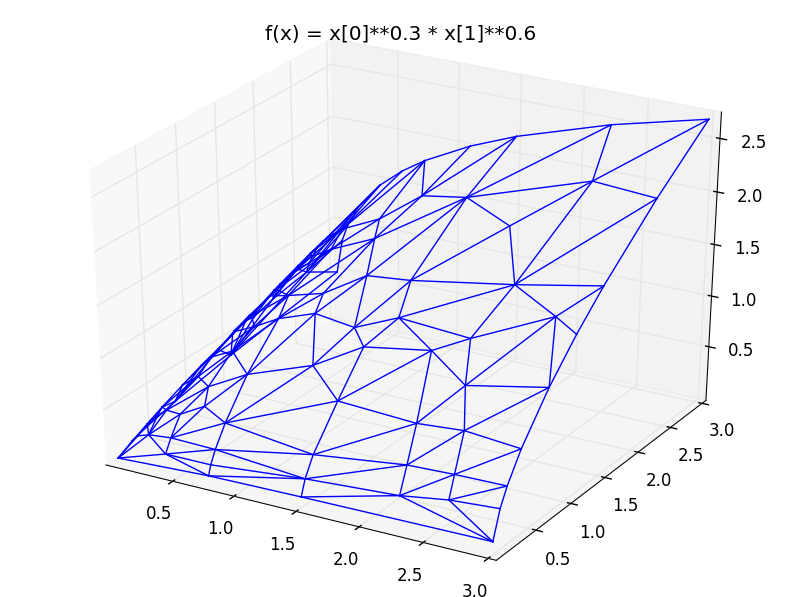View on GitHub

# Delaunay linterp

## n-dimensional piecewise linear interpolation using Delaunay triangulation in C++

Project page: http://rncarpio.github.com/delaunay_linterp

### What is `delaunay_linterp`?

`delaunay_linterp` is a C++ header-only library for N-dimensional piecewise linear interpolation of unstructured data, similar to Matlab's griddata and SciPy's griddata commands. Suppose we are given a set of data points `(x, f(x))` where `x` is N-dimensional. The idea is to construct an N-dimensional Delaunay triangulation of the `x` coordinates of the data points; each vertex in the triangulation corresponds to a data point `(x, f(x))`. To compute an interpolated value for `xi`, we:

• locate the simplex containing `xi`
• calculate the barycentric coordinates of `xi` in the simplex
• calculate `interp(xi)` as a weighted sum of the values of `f(x)` at the vertices of the simplex

The interpolated surface is linear within each simplex of the triangulation.

The CGAL computational geometry library is used for the underlying triangulation code. Other libraries that are used are:

• Boost - C++ libraries
• GMP - arbitrary precision arithmetic (on Windows, MPIR is used)
• MPFR - multi-precision floating point
• Eigen - C++ linear algebra

Points can be added incrementally. In addition, a function specifying `f(x)` can be passed to the triangulation. `delaunay_linterp` can adaptively choose where to insert data points, by maintaining a list of approximation errors associated with each simplex of the triangulation. This allows a good approximation of arbitrary functions with few data points, compared to regular grids.

### dD vs. 2D

CGAL has more than one package for constructing a Delaunay triangulation:

• a d-dimensional library that uses the lifting transform, then constructs a convex hull in d+1 dimensions. This is very flexible and can handle arbitrary dimensions, but it is much slower than the libraries specifically designed for 2D and 3D.
• 2D and 3D specific libraries which are much faster.

`delaunay_linterp` provides classes using the dD and 2D libraries (3D coming soon...).

• for dD, use the `delaunay_d_interp` class. Caveats: currently, it cannot handle interpolation queries outside the convex hull of the inserted points
• for 2D, use the `delaunay_2_interp` class

### C++ interface

Here is an example in C++:

```#include <ctime>
#include "delaunay_2_interp.h"

// return an evenly spaced 1-d grid of doubles.
std::vector<double> linspace(double first, double last, int len) {
std::vector<double> result(len);
double step = (last-first) / (len - 1);
for (int i=0; i<len; i++) { result[i] = first + i*step; }
return result;
}

// the function to interpolate.
double fn (double x1, double x2) { return pow(x1,0.3) * pow(x2,0.6); }

int main(int argc, char **argv) {
int n_points = 10;
clock_t t1, t2;

// first, try a rectangular grid
Delaunay_incremental_interp_2 triang;
std::vector<double> grid = linspace(0., 5., n_points);
t1 = clock();
for (int i=0; i<n_points; i++) {
for (int j=0; j<n_points; j++) {
array<double,2> args = {grid[i], grid[j]};
triang.insert(args.begin(), args.end(), fn(args, args));
}
}
t2 = clock();
printf("regular grid: %d insertions, %d clocks, %f sec\n", n_points*n_points, (t2-t1), ((double)(t2 - t1)) / CLOCKS_PER_SEC);

// second, try adaptive point placement
std::function<double(double, double)> fn_obj(fn);
// insert boundary points
array<double,2> args;
t1 = clock();
args=grid.front(); args=grid.front();   adaptive_triang.insert(args.begin(), args.end(), fn(args, args));
args=grid.front(); args=grid.back();    adaptive_triang.insert(args.begin(), args.end(), fn(args, args));
args=grid.back(); args=grid.front();    adaptive_triang.insert(args.begin(), args.end(), fn(args, args));
args=grid.back(); args=grid.back();     adaptive_triang.insert(args.begin(), args.end(), fn(args, args));
for (int i=0; i<n_points*n_points-4; i++) {
}
t2 = clock();
printf("adaptive grid: %d insertions, %d clocks, %f sec\n", n_points*n_points, (t2-t1), ((double)(t2 - t1)) / CLOCKS_PER_SEC);

// compare interpolated value vs. actual function
std::vector<double> true_f_vals, interp_grid = linspace(0., 5., 2*n_points);
for (int i=0; i<interp_grid.size(); i++) {
for (int j=0; j<interp_grid.size(); j++) {
array<double,2> args = {interp_grid[i], interp_grid[j]};
true_f_vals.push_back(fn(args, args));
}
}

// get the interpolated values
t1 = clock();
for (int i=0; i<interp_grid.size(); i++) {
for (int j=0; j<interp_grid.size(); j++) {
array<double,2> args = {interp_grid[i], interp_grid[j]};
regular_triang_vals.push_back(triang.interp(args.begin(), args.end()));
}
}
t2 = clock();
printf("regular grid: %d interpolations, %d clocks, %f sec\n", regular_triang_vals.size(), (t2-t1), ((double)(t2 - t1)) / CLOCKS_PER_SEC);
t1 = clock();
for (int i=0; i<interp_grid.size(); i++) {
for (int j=0; j<interp_grid.size(); j++) {
array<double,2> args = {interp_grid[i], interp_grid[j]};
}
}
t2 = clock();
printf("adaptive grid: %d interpolations, %d clocks, %f sec\n", adaptive_triang_vals.size(), (t2-t1), ((double)(t2 - t1)) / CLOCKS_PER_SEC);

// compute sum of squared errors
double sse1=0.0, sse2=0.0, diff;
for (int i=0; i<true_f_vals.size(); i++) {
diff = true_f_vals[i] - regular_triang_vals[i];
sse1 += diff*diff;
sse2 += diff*diff;
}
printf("regular grid: sum of squared errors: %f\n", sse1);
printf("adaptive grid: sum of squared errors: %f\n", sse2);

return 0;
}
```

produces:

``````regular grid: 100 insertions, 6 clocks, 0.006000 sec
adaptive grid: 100 insertions, 11 clocks, 0.011000 sec
regular grid: 400 interpolations, 2 clocks, 0.002000 sec
adaptive grid: 400 interpolations, 2 clocks, 0.002000 sec
regular grid: sum of squared errors: 5.800002
adaptive grid: sum of squared errors: 0.044019
``````

### Python interface

A Python interface is provided, using Andreas Klöckner's pyublas library. Pre-built binaries are in the `windows_binaries` directory; these were built against PythonXY version 2.7.3.1.

Example:

```import scipy
import matplotlib.pyplot as plt
from mpl_toolkits.mplot3d import Axes3D
import _delaunay_2_python as _delaunay2

def plot_triang_2d(triang, title=None, show_concave=False):
fig = plt.figure()
ax = Axes3D(fig)
(all_segments, min_coords, max_coords) = triang.get_line_segments()

for segment in all_segments:
(p0, p1, is_concave) = segment
if (show_concave):
color = ('b' if (is_concave) else 'r')
else:
color = 'b'
(xpair, ypair, zpair) = zip(p0, p1)
ax.plot3D(xpair, ypair, zpair, color=color)
ax.set_xlim(min_coords, max_coords)
ax.set_ylim(min_coords, max_coords)
ax.set_zlim(min_coords, max_coords)
if (title != None):
ax.set_title(title)
return ax

# the function to be approximated
def fn(x):
return x**0.3 * x**0.6

# create triangulation object
triang = _delaunay2.DelaunayInterp2(fn)

# insert boundary points
for x in [ [0.01, 0.01], [0.01, 3.0], [3.0, 0.01], [3.0, 3.0] ]:
triang.insert(x, fn(x))

for i in range(100):
triang.insert_largest_error_point()

# plot triangulation
plot_triang_2d(triang, title="f(x) = x**0.3 * x**0.6")
```

produces